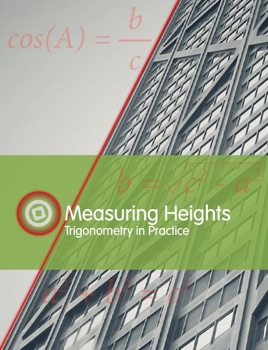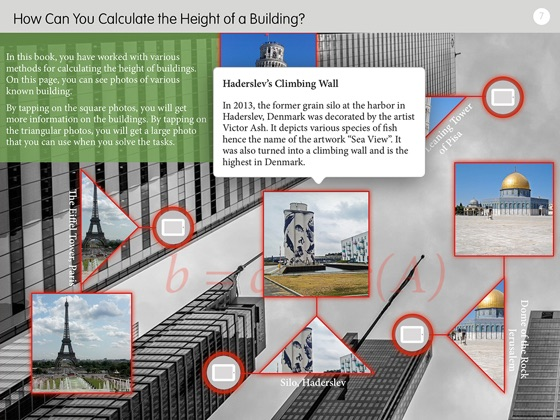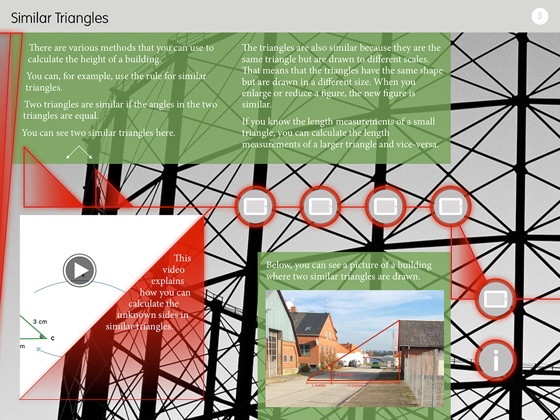• \$0.99

## Publisher Description

Is it possible to calculate the height of a building by using just a stick and a tape measure? What do surveyors use when they need to calculate a specific height? What is trigonometry? How can trigonometry give us tools to be able to calculate the height of a building?

This book works with similar triangles including what the magnification factor and the reduction factor are and how they can be used to calculate height. It also works with the law of cosine and solving equations. The reader is asked to critically evaluate their measurements and consider what the cause of a deviation from the actual height might be due to.

The book proposes an active approach to mathematics teaching where the reader through practice, tests different methods for calculating the height of various buildings. Likewise, it involves the use of various apps to illustrate, explain and work out the assigned tasks.

The book is designed to be used from age 12 through adult education as well as in interdisciplinary courses and other projects involving mathematics.

•••